# PSAT Math : How to find the perimeter of a rhombus

## Example Questions

### Example Question #1 : How To Find The Perimeter Of A Rhombus

If the area of a rhombus is 24 and one diagonal length is 6, find the perimeter of the rhombus.

8

16

20

24

12

20

Explanation:

The area of a rhombus is found by

A = 1/2(d1)(d2)

where d1 and d2 are the lengths of the diagonals.  Substituting for the given values yields

24 = 1/2(d1)(6)

24 = 3(d1)

8 = d1

Now, use the facts that diagonals are perpendicular in a rhombus, diagonals bisect each other in a rhombus, and the Pythagorean Theorem to determine that the two diagonals form 4 right triangles with leg lengths of 3 and 4.  Since 32 + 42 = 52, each side length is 5, so the perimeter is 5(4) = 20.

### Example Question #1 : Quadrilaterals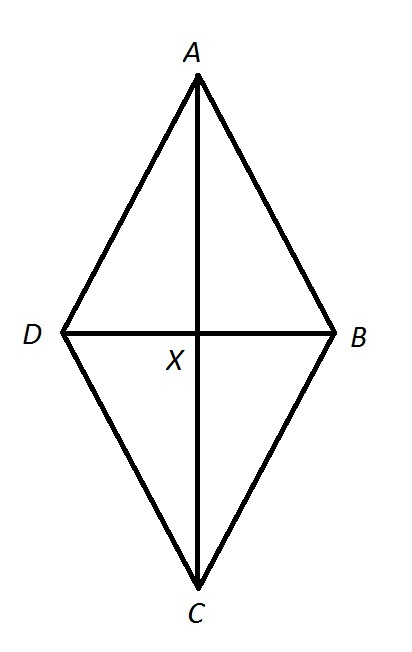Note: Figure NOT drawn to scale.

Calculate the perimeter of Quadrilateralin the above diagram if: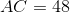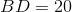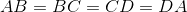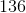Insufficient information is given to answer the question.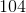Explanation:, so Quadrilateralis a rhombus. Its diagonals are therefore perpendicular to each other, and the four triangles they form are right triangles. Therefore, the Pythagorean theorem can be used to determine the common sidelength of Quadrilateral.

We focus on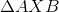. The diagonals are also each other's bisector, so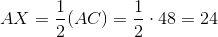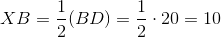By the Pythagorean Theorem,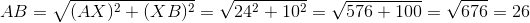26 is the common length of the four sides of Quadrilateral, so its perimeter is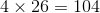.